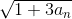### Create an Account

Home / Questions / When defining sequence {an} as a1=5, an+1=\sqrt{1+3a_{n}} Show a convergence of a and fin...

# When defining sequence {an} as a1=5, an+1=\sqrt{1+3a_{n}} Show a convergence of a and find its limit.

When defining sequence {an} as a1=5, an+1=Show a convergence of a and find its limit.

Apr 21 2021 View more View LessSubscribe To Get Solution